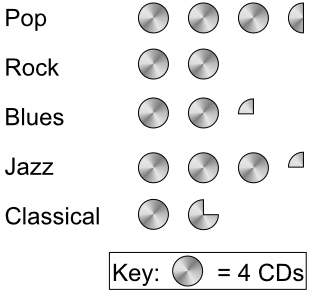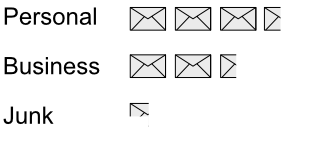Pictograms

## Pictograms

A Pictogram uses simple pictures to represent data. The total number of items in a set of data is shown by a number of pictures, where each picture represents one or more items.

An individual picture can be subdivided. If a picture represents four items, then a quarter of a picture represents one item.

A pictogram will always have a key, which indicates how many items each picture on the pictogram represents.

## Example 1

The pictogram shows the number of CDs sold at a charity event. How many pop CDs were sold?Each CD is worth 4. There are three full and one frac(1)(2) CD.

4 x 3frac(1)(2) = 14 CDs

## Example 2

The pictogram shows emails received over a day. The key to the pictogram has been left off the diagram. How many emails does each envelope represent, if 28 personal emails were received?3frac(1)(2) envelopes = 28 emails

Let x = number of emails in an envelope

Change 3frac(1)(2) to a top-heavy fraction = frac(7)(2)

frac(7)(2)x = 28

x = 8